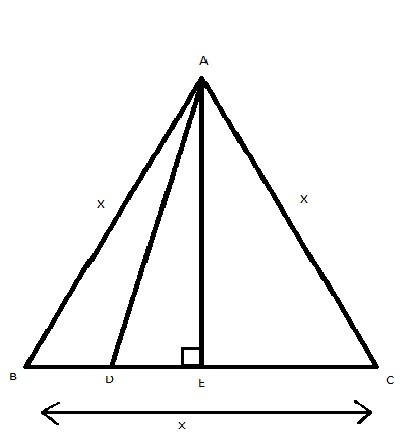QUESTION

# In a equilateral $\Delta ABC$, $D$ is a point on $BC$ such that $BD = \dfrac{1}{3}BC$.Prove that $9{\left( {AD} \right)^2} = 7{\left( {AB} \right)^2}$.

Hint: First of all, construct a perpendicular in $\Delta ABC$. Proof that the triangles formed by this perpendicular are equal by using RHS congruence. Then use Pythagoras’s theorem to prove the given statement. So, use this concept to reach the solution of the given problem.

Given in equilateral $\Delta ABC$, $D$ is a point on $BC$ such that $BD = \dfrac{1}{3}BC$.
Let draw $AE \bot BC$ in $\Delta ABC$, as shown in the below figure:Since all sides of an equilateral triangle is equal, we have
$AB = BC = AC$
Let $AB = BC = AC = x$
Given $BD = \dfrac{1}{3}BC$
$\Rightarrow BD = \dfrac{1}{3}x$
In $\Delta AEB$ and $\Delta AEC$
$\Rightarrow AE = AE{\text{ }}\left[ {{\text{common}}} \right] \\ \Rightarrow AB = AC{\text{ }}\left[ {{\text{Both }}x{\text{ as it is equilateral triangle}}} \right] \\ \Rightarrow \angle AEB = \angle AEC{\text{ }}\left[ {{\text{Both 9}}{{\text{0}}^0}{\text{ as }}AE \bot BC} \right] \\$
Hence by RHS congruency
$\Delta AEB \cong \Delta AEC$
By common property of congruence triangle, we have $BE = EC$
So, $BE = EC = \dfrac{1}{2}BC$
$BE = EC = \dfrac{1}{2}x{\text{ }}\left[ {\because BC = x} \right]$
So, we have
$\Rightarrow BE = \dfrac{x}{2} \\ \Rightarrow BD + DE = \dfrac{x}{2} \\ \Rightarrow \dfrac{x}{3} + DE = \dfrac{x}{2} \\ \Rightarrow DE = \dfrac{x}{2} - \dfrac{x}{3} = \dfrac{{3x - 2x}}{6} \\ \therefore DE = \dfrac{x}{6} \\$
Using Pythagoras theorem i.e.,
${\left( {{\text{Hypotenuse}}} \right)^2} = {\left( {{\text{Height}}} \right)^2} + {\left( {{\text{Base}}} \right)^2}$
Now in right $\Delta AEB$
$\Rightarrow A{B^2} = A{E^2} + B{E^2} \\ \Rightarrow {x^2} = A{E^2} + {\left( {\dfrac{x}{2}} \right)^2} \\ \Rightarrow A{E^2} = {x^2} - \dfrac{{{x^2}}}{4} \\ \therefore A{E^2} = \dfrac{{3{x^2}}}{4}........................................................\left( 1 \right) \\$
Similarly, in right $\Delta AED$ using Pythagoras’s theorem we have
$\Rightarrow A{D^2} = A{E^2} + D{E^2} \\ \Rightarrow A{D^2} = \dfrac{{3{x^2}}}{4} + {\left( {\dfrac{x}{6}} \right)^2}{\text{ }}\left[ {{\text{From 1}}} \right] \\ \Rightarrow A{D^2} = \dfrac{{27{x^2} + {x^2}}}{{36}} \\ \Rightarrow A{D^2} = \dfrac{{7{x^2}}}{9} \\ \therefore 9A{D^2} = 7A{B^2}{\text{ }}\left[ {\because AB = x} \right] \\$
Hence proved.
Note: Pythagoras’s theorem states that “In a right-angled triangle, the square of the hypotenuse side is equal to the sum of the squares of the other two sides”. In two right-angled triangles, if the length of the hypotenuse and one side of one triangle is equal to the length of the other triangle, then the two triangles are congruent.